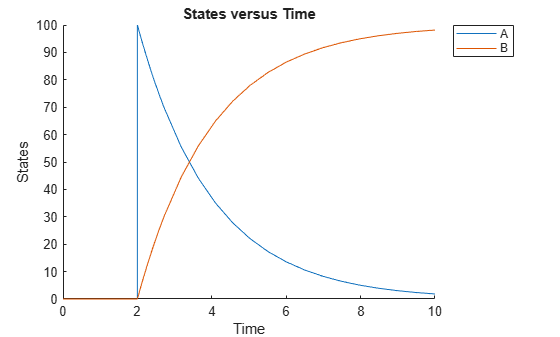Add event object to model object

## Syntax

```eventObj = addevent(modelObj, 'TriggerValue', 'EventFcnsValue') eventObj = addevent(...'PropertyName', PropertyValue...) ```

## Arguments

 `modelObj` `Model object`. `TriggerValue` Required property to specify a trigger condition. Must be a MATLAB® expression that evaluates to a logical value. Use the keyword `'time'` to specify that an event occurs at a specific time during the simulation. For more information, see `Trigger`. `EventFcnsValue` Character vector or a cell array of character vectors, each of which specifies an assignment of the form `'objectname = expression'`, where `objectname` is the name of a valid object. Defines what occurs when the event is triggered. For more information, see `EventFcns`. `PropertyName` Property name for an event object from Property Summary. `PropertyValue` Property value. For more information on property values, see the property reference for each property listed in Property Summary.

## Description

```eventObj = addevent(modelObj, 'TriggerValue', 'EventFcnsValue')``` creates an `Event object` (`eventObj`) and adds the event to the model (`modelObj`). In the event object, this method assigns a value (`TriggerValue`) to the property `TriggerCondition`, assigns a value (`EventFcnsValue`) to the property `EventFcns`, and assigns the `model object` (`modelObj`) to the property `Parent`. In the model object, this method appends the event object to the property `Events`.

When the trigger expression in the property `Trigger` changes from false to true, the assignments in `EventFcns` are executed during simulation.

For details on how events are handled during a simulation, see Events in SimBiology Models.

``` eventObj = addevent(...'PropertyName', PropertyValue...)``` defines optional properties. The property name and property value pairs can be any format supported by the function `set`.

## Property Summary

 Active Indicate object in use during simulation EventFcns Event expression Name Specify name of object Notes HTML text describing SimBiology object Parent Indicate parent object Tag Specify label for SimBiology object Trigger Event trigger Type Display SimBiology object type UserData Specify data to associate with object

## Examples

collapse all

This example shows how to add an event to a SimBiology model.

Create a simple model with a mass action reaction `A -> B`, where A and B are species. Also add the reaction rate parameter, p1, with the parameter value of 0.5.

```model = sbiomodel('example'); r1 = addreaction(model,'A -> B'); kl = addkineticlaw(r1,'MassAction'); p1 = addparameter(model,'p1',0.5); kl.ParameterVariableNames = 'p1';```

Increase the amount of species A to 100 at time = 2. You can do this by adding an event object to the model. You must specify the event trigger (`time >= 2`), and also the event function, which defines what happens when the event is triggered. In this example, the event function is `A = 100`.

`e1 = addevent(model,'time>=2','A = 100');`

Simulate the model, and plot the result.

```sd = sbiosimulate(model); sbioplot(sd);```### Topics

Introduced in R2007b

## SupportGet trial now